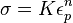# Problem 8053. Stress-Strain Properties - 6

The total energy absorbed by a material up to failure in a tensile test is termed the absorbed strain energy. With respect to the figure below, it is the total area of the elastic and plastic regions and can be calculated by integrating the stress-strain curve. As a first approximation, many stress-strain responses can be approximated by:where K is a strength coefficient, eps_p is the plastic strain, and n is the hardening exponent. Stress as a function of strain can be calculated by creating a strain vector from zero to the ultimate strain and integrating the stress values in that vector.(from simsolid.com)
Write a function to return the absorbed strain energy for a material provided K and n. If the material does not strain harden, then K and n will be set equal to zero. In these cases, the absorbed strain energy is equal to the resilience (triangular area up to yield point) and any absorbed plastic energy, if applicable, which can be approximated by a rectangle from the yield point to the failure point with those stresses being equal. If the ultimate strain equals the yield strain, that rectangular area is zero.
Previous problem: 5 - stiffness-to-weight ratio. Next problem: 7 - toughness.

### Solution Stats

23.75% Correct | 76.25% Incorrect
Last Solution submitted on Nov 04, 2023

### Community Treasure Hunt

Find the treasures in MATLAB Central and discover how the community can help you!

Start Hunting!